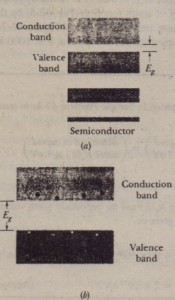# Semiconductors

Semiconductors

semiconductor is like that of an insulator. The main difference is that the semiconductor has a much smaller energy gap EI/ between the top of the highest filled band (called the valence band) and the bottom of the vacant band just above it (called  the conduction band). Thus, there is no doubt that silicon (Ell = 1.1 eV) is a semiconductor and diamond (Ell = 5.5 eV) is an insulator. In silicon-but not in diamond-there is a real possibility that thermal agitation at room  temperature will  cause electrons to jump the gap from the valence band to the conduction band. In we compared three basic electrical properties of copper, our prototype metallic conductor, and silicon, our prototype semiconductor. Let us  look again at that table, one row at a time, to see how a semiconductor differs from metal.Number Density of Charge Carriers n

The bottom row of Table 42-1 shows that copper has far more charge carriers per unit volume than silicon. by a factor of about 1013. For copper, each atom contributes one electron, its single valence electron, to the conduction process. Charge carriers  in silicon arise only because, at thermal equilibrium, thermal agitation causes a certain (very small) number of valence-band electrons to jump the energy gap into the conduction band, leaving an equal number of unoccupied energy states, called  holes, in the valence band. Figure 42-Sh shows the situation.Both the electrons in the conduction band and the holes in the valence band serve as charge carriers. The holes do so by permitting a certain freedom of movement to electrons in the valence band that, in the absence of holes, would be gridlocked.  If an electric field E is set up in a semiconductor, the electrons J.n the valence band, being negatively charged, tend to drift in the direction opposite E. This causes the positions of the holes to drift in the direction of E. In effect, the holes behave  like moving particles of charge +e.It may help to think of a row of cars parked bumper to bumper, with the leading car at one car’s length from a barrier. If the leading car moves forward to the barrier, it opens up a car’s length space behind it. The second car can then move up to fill that space, allowing the third car to move up, and so on. The motions of the many cars toward the barrier are most simply analyzed by focusing attention on the drift of the single “hole” (parking space) away from the barriern-Type Semiconductors

The electronics in ilIl isolated silicon atom are arranged in subshells according to the scheme

in which, as usual, the. superscripts (which allied to 14, the atomic number of silicon) represent the numbers of electrons in the specified subshells, Figure 42-9a is a flattened-out representation of a pertinent of the lattice of pure
silicon in which the portion has J.ee  projecte4 ont8 a ,Jane; compare the figure with  which represents the unit cell of the lattice in three lIimensions. Each SiliCORatom c contributes its pair of 3s electrons and its pair of 3p electrons to form  a rigid two-electron covalent “nd with each ef its four nearest neighbors. (A covalent bond is a link between two atoms in which the atoms share a pair of electrons.) The four atoms that lie within the unit cell in  show these four bonds.  The electrons that form the silicon-silicon bonds constitute the 1’llle1¥’eHnjof the silicon s.mplt. U an e\ectrot\ is tom ftOm one of these ~ends so that it some free to wander throughout the lattice, we say that the electron has been raised from the valence band to the conduction band. The minimum energy required  to do this the gap energy ER• •  because fo ur of its electrons are involved in bonds, each silicon “atom” is actually, an ion consisting of an inert unlike electron cloud (containing IO electrons) surrounding a nucleus whose charge is + I4#!, where 14 is the atomic number of silicon. The net charge of each of these ions is thus +4e, and the ions are said to  have a valence number of 4. _In Fig. 42-9b the central silicon ion has been replaced by an  Iris(valence = 5). Four of the valence ele  trens of the phosphorus form bonds with the four surrounding silicon ions. The fifth (“extra”) electron is only loosely bound to  the phosphorus ion core. On an energy-band diagram, we usually .say that such an electron occupies a localized energy state that lies within the energy gap, at average energy interval Ed bel,w the  nnom of the conduction band; this is indicated inFig. 42-100. Because Ed ~ ER’ the energy required to excite electrons from themselves into the conduction .and is  much Jess than tat required te excite silicon valence electrons into the conduction band.  The phosphorus atom is called a donor atom because it readily donates an electron to the conduction band. In fact, at room temperature virtually DII the electronic tribute by the donor atoms are in the conduction band. By adding donor atoms, it is possible to increase greatly the number of electrons in the conduction band, by a factor very much larger than Fig. 42-100 suggests, Semiconductors doped with «!«meratoms are called II-type \$emic englut:tors; then stands for negative, to imply that the negative charge carriers introduce4 into the conduction band greatly outnumber the positive charge carriers, which are the holes.